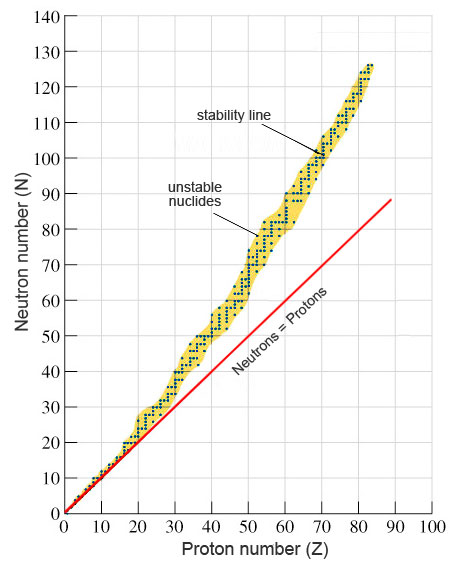## Stable and Unstable Nuclei

An atom is electrically neutral. It contains an equal number of positively charged protons and negatively charged electrons and their charges balance. The nucleus however contains only positively charged protons which are closely packed together in a very small volume (remember neutrons have no charge). From the laws of physics (Coulomb’s Law) one would expect that the protons being of the same charge and so close together would exert strong repulsive forces on each other. The combined gravitational force from the protons and neutrons in a nucleus is insignificant as an attractive force because their masses are so tiny. This implies there must be an additional attractive force similar in size to the electrostatic repulsion which holds the nucleus together.

## Strong Nuclear Force

A strong force must exist between the protons and neutrons in a nucleus to overcome the electrostatic repulsion between the protons. This additional force is called the strong nuclear force. This force is attractive over short distances, but this is not all. If the force was only attractive then it would pull the protons and neutrons together to an ever decreasing small volume of space. We know this not to be the case as each nucleus contains a distinct number of protons and neutrons which retain their identity. The more protons and neutrons, the bigger the nucleus and thus the separation between the protons and neutrons in a nucleus must be a fixed distance. This means the strong nuclear force must be repulsive at a very small range as well as being attractive over a slightly larger distance. This is the force that holds the nucleus together and the energy associated with this force is called the binding energy.

It is the amount of strong nuclear force and the associated binding energy in a nucleus that determines whether it will be stable or unstable.

### Stable Nuclei

A stable atom is an atom that has enough binding energy to hold the nucleus together permanently. Many nuclei in nature are very stable, most of the nuclei formed at the creation of the universe or after supernovae explosions many millions of years ago are still in existence now.

The graph below is a plot of neutron number against proton number. It is used as rule to determine which nuclei are stable or unstable.Nuclei which lie on the stability line are stable nuclei. From the plot it can be seen that many of the stable nuclei have equal number of protons and neutrons. These are usually the elements in the lighter section of the periodic table. For the heavier stable nuclei the there are approximately 50% more neutrons to protons.

### Unstable Nuclei

In unstable nuclei the strong nuclear forces do not generate enough binding energy to hold the nucleus together permanently. It is unstable nuclei that are radioactive and are referred to as radioactive nuclei and in the case of their isotopes called radioisotopes.

The unstable nuclei lie above and below the line of stability in the neutron – proton plot. This gives information of the type of radioactive decay they will undergo. Nuclei which lie above the line of stability contain too many neutrons to be stable. They are referred to as “neutron rich”. Those that lie below the line of stability contain too many protons to be stable and are called “proton rich”.

In summary it is the balance of protons and neutrons in a nucleus which determines whether a nucleus will be stable or unstable. Too many neutrons or protons upset this balance disrupting the binding energy from the strong nuclear forces making the nucleus unstable. An unstable nucleus tries to achieve a balanced state by given off a neutron or proton and this is done via radioactive decay.